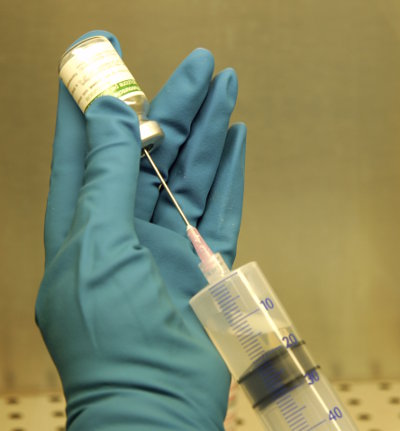# Divide Decimals

## Dividing Decimals by Powers of Ten:

If you divide a decimal number by 10, the decimal point moves 1 place to make the number smaller
(for example 2345.6 ÷ 10 = 234.56).

If you divide a decimal number by 100, the decimal point moves 2 places to make the number smaller
(for example 2345.6 ÷ 100 = 23.456).

If you divide a decimal number by 1000, the decimal point moves 3 places to make the number smaller
(for example 2345.6 ÷ 1000 = 2.3456).

## Example One - Price of Strawberries3 kilograms of strawberries cost \$19.65. What is the price of one kilogram?

\$19.65 ÷ 3 = \$6.55

## Questions - Price of Food

Q1. A dozen eggs (12 eggs) cost \$5.16. What is the price of one egg?
Q2. 10 apples cost \$4.90. What is the cost of one apple?
Q3. 5 bags of Persian Cotton Candy cost \$24.25. What does one bag cost?

A1. 43 cents
A2. 49 cents
A3. \$4.85

## Example Two - Injection DosesA glass vial contains 45.5 millilitres of medicine. Each injection requires 0.5 millilitres. How many injections can be given from one glass vial?

 = 45.5 0.5
 = 455 (Multiply both the numerator and the denominator by 10 to get rid of the decimal in the denominator.) 5

= 91 injections

## Example Three - Smaller Injection Doses

Using the same-sized glass vial containing 45.5 millilitres of medicine, each injection has 0.05 millilitres. How many injections can be given from one glass vial?

 = 45.5 0.05
 = 4550 (Multiply both the numerator and the denominator by 100 to get rid of the decimal in the denominator.) 5

= 910 injections

(a) \$46.80
(b) \$10.80
(c) \$20.70?

(a) 52
(b) 12
(c) 23

## Maths Fun - Dividing by Zero

Try dividing any number by zero on your calculator. The calculator will display and "Error" message because dividing by zero is impossible. However, we can approximate what number we could get if we divided a number such as "8" by numbers that are close to zero.

Use your calculator to work out the following:

• 8 ÷ 1
• 8 ÷ 0.1
• 8 ÷ 0.01
• 8 ÷ 0.001
• 8 ÷ 0.0001
• 8 ÷ 0.000000000000000000000000000000000001

What do you notice?